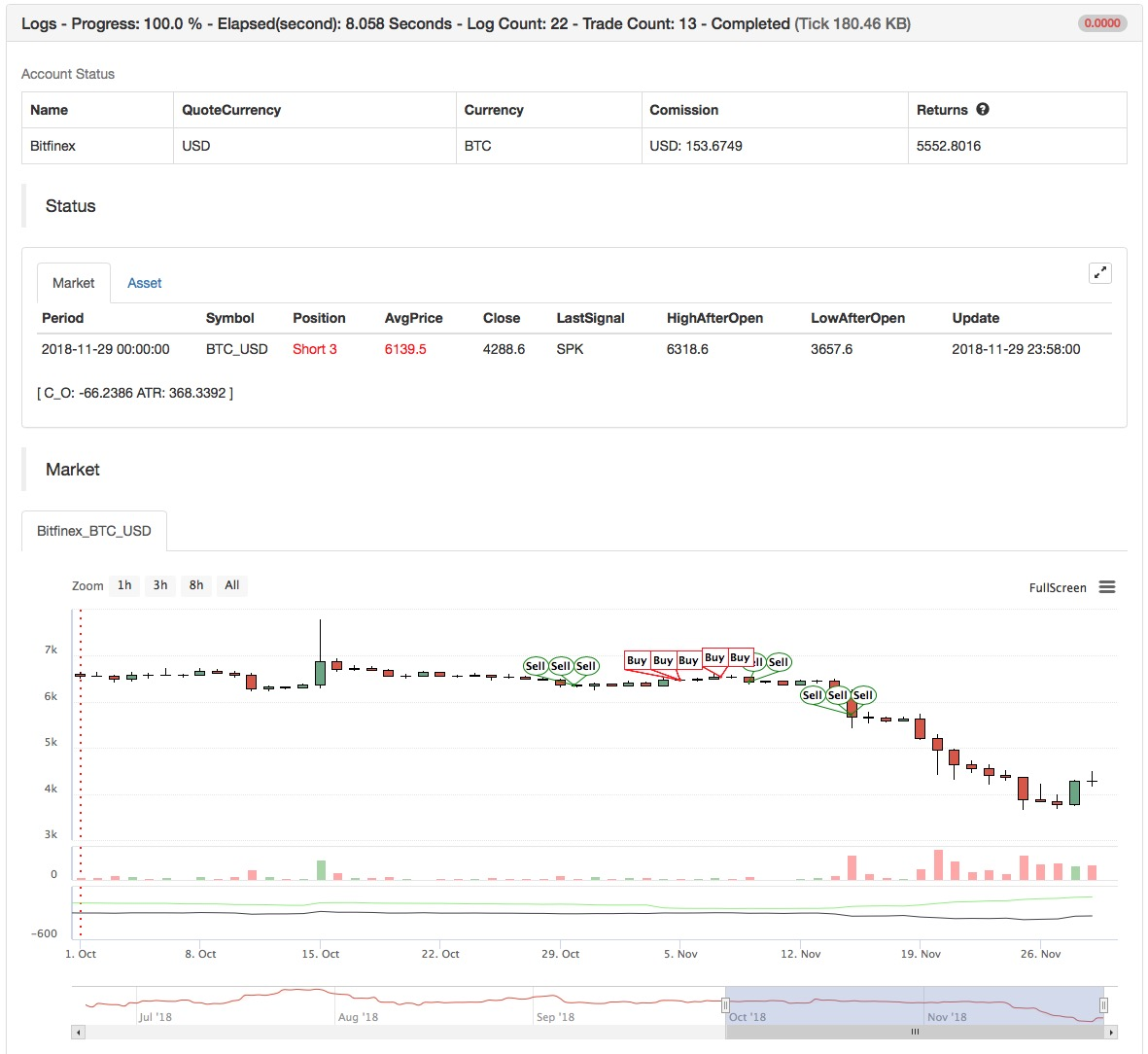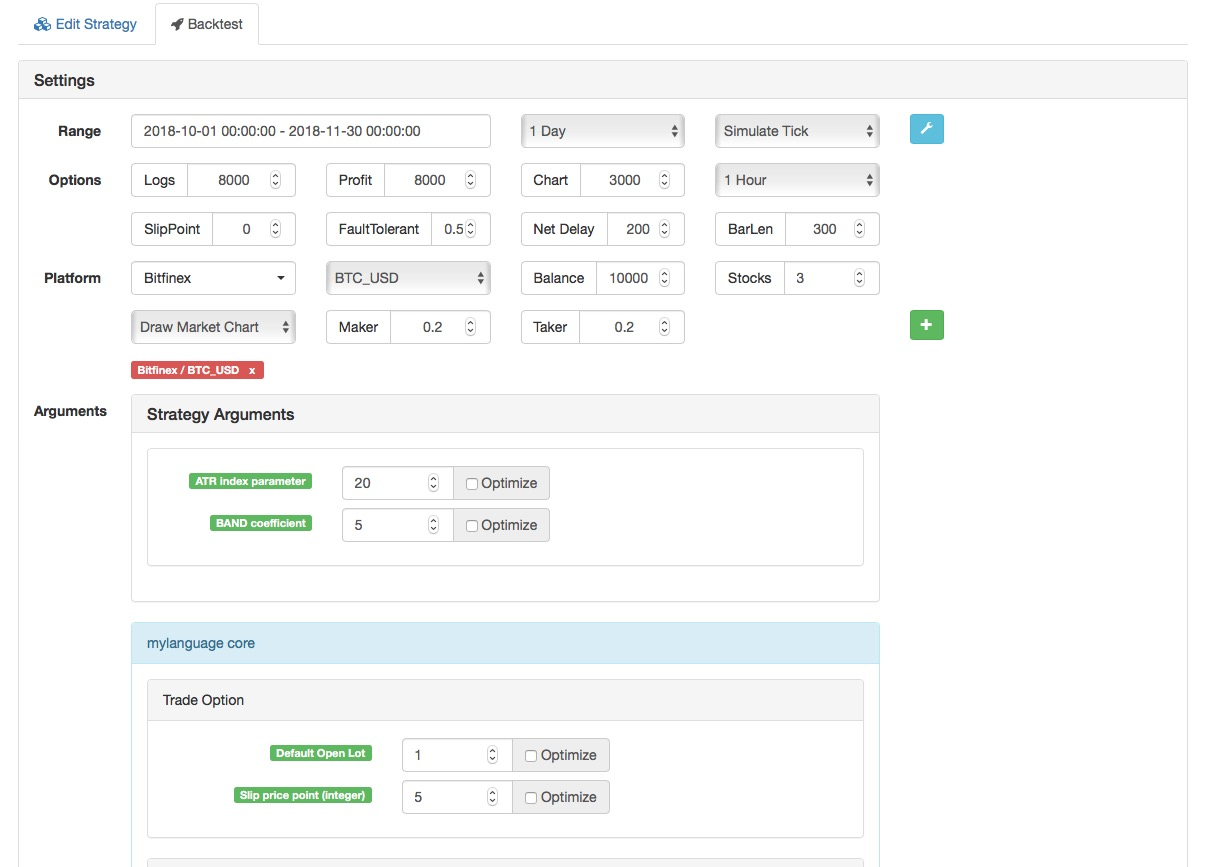# The average of difference - combined with ATR

Author: littleDreamXX, Date: 2018-11-30 09:25:07
Tags: Commodity-futures ATR MyLanguage

• Enter: The average of difference of opening price minus closing price determines the trend combined with ATR, the entry point is also the exit point in reverse;

• Exit: Stop-loss point should be fixed at the opening of the position, and if there is a reverse trend in price, close the position.

• Data Cycle: 1 Day

• Data contract: index contract

• Transaction contract: main contract• Main chart: none

• Secondary chart: ATR, formula ATR ^^ MA (TR, N); C_O, formula C_O: EMA (C, N) - EMA (O, N);

```(*backtest
start: 2018-10-01 00:00:00
end: 2018-11-30 00:00:00
period: 1d
exchanges: [{"eid":"Futures_CTP","currency":"FUTURES","balance":20000}]
*)

// 变量
// variable

LOTS:=MAX(1,INTPART(MONEYTOT/(O*UNIT*0.1)));
C_O:EMA(C,N)-EMA(O,N);
B:=CROSSUP(C_O,0);
S:=CROSSDOWN(C_O,0);
TR:=MAX(MAX((H-L),ABS(REF(C,1)-H)),ABS(REF(C,1)-L));
ATR:MA(TR,N);
BAND:=ATR*0.1*M;
PRICE_BPK:=VALUEWHEN(B,H+BAND);
PRICE_SP:=VALUEWHEN(B,L-BAND);
PRICE_SPK:=VALUEWHEN(S,L-BAND);
PRICE_BP:=VALUEWHEN(S,H+BAND);

// 策略逻辑
// strategy logic
BARPOS>N AND C_O>0  AND C>=PRICE_BPK,BPK(LOTS);
BARPOS>N AND C_O<0  AND C<=PRICE_SPK,SPK(LOTS);

// 下单
// place an order
S,SP(BKVOL);
B,BP(SKVOL);

C<=PRICE_SP,SP(BKVOL);
C>=PRICE_BP,BP(SKVOL);
```

Related

More